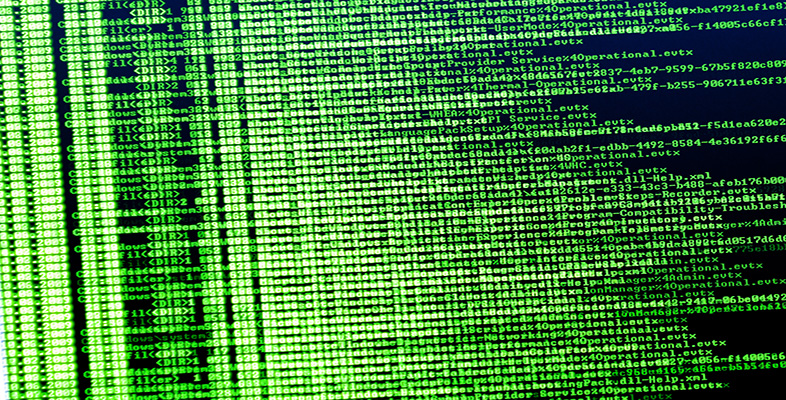Exploring data: Graphs and numerical summaries

Start this free course now. Just create an account and sign in. Enrol and complete the course for a free statement of participation or digital badge if available.

Free course

# 4.4 Histograms and scatterplots: summary

Two common graphical displays, most frequently used for continuous data (arising from measurements), have been introduced in this section. A histogram is in a sense a development of the idea of a bar chart. A set of continuous data is divided up into groups, the frequencies in the groups are found, and a histogram is produced by drawing vertical bars, without gaps between them, whose heights are proportional to the frequencies in the groups. You have seen that the shape of a histogram drawn from a particular data set can depend on the choices made for the grouping of the data.

Scatterplots represent the relationship between two variables. The variables generally have to be numerical, and are commonly continuous, though they may also be discrete (counted). One variable is plotted on the horizontal axis and the other on the vertical axis. One point is plotted, in the appropriate position, for each individual entity (person, animal, country) in the data set. As well as making it easy to identify any general pattern, such as a straight line, in the relationship between the variables, a scatterplot can help in the identification of outliers. These are data points that lie a long way from the general pattern in the data. In some cases, the patterns shown in a scatterplot can be made clearer by omitting an outlier, though this is very often not an advisable thing to do. In other cases, it may help to transform the data by applying some appropriate function to one or both of the variables involved.

M248_1